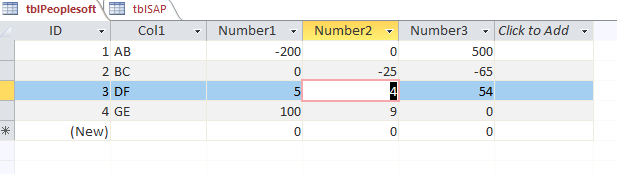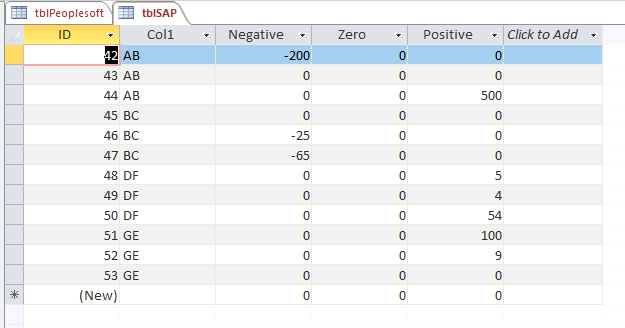Peoplesoft To SAP Migration Via Access

I received an interesting question yesterday from a potential customer.

They are migrating all their Oracle PeopleSoft data to SAP.

Basically, how to take 1 (PeopleSoft) row and make it into multiple rows (SAP)?

For example:

PeopleSoft format: A B -200 0 500

to SAP format:

A B -200 0 0
A B 0 0 0
A B 0 0 500

So I decided to make a blog post on it so others may benefit:

Here is what the source would look like:And here is what the result would look like:…and then we’ll save the table to a csv file to upload to SAP.

Here is the code to get the data into the tblSAP:

Sub SAPFormat()
Dim rst As Recordset
Dim strInsSQL As String
Dim intCounter As Integer

Dim dblNegative As Double
Dim dblZero As Double
Dim dblPositive As Double

Set rst = CurrentDb.OpenRecordset("SELECT Col1, Number1,Number2,Number3 FROM tblPeopleSoft")

CurrentDb.Execute "DELETE * FROM tblSAP"

rst.MoveFirst
Do Until rst.EOF

For intCounter = 1 To 3
'reset the variables
dblNegative = 0
dblZero = 0
dblPositive = 0

'evaluate the numbers
Select Case intCounter
Case 1 'evaluate number 1
If rst("Number1") < 0 Then
dblNegative = rst("Number1")
dblZero = 0
dblPositive = 0
End If
If rst("Number1") = 0 Then
dblNegative = 0
dblZero = rst("Number1")
dblPositive = 0
End If
If rst("Number1") > 0 Then
dblNegative = 0
dblZero = 0
dblPositive = rst("Number1")
End If

'stay in the loop but insert the record
GoTo InsertThis
Case 2 'evaluate number 2
If rst("Number2") < 0 Then
dblNegative = rst("Number2")
dblZero = 0
dblPositive = 0
End If
If rst("Number2") = 0 Then
dblNegative = 0
dblZero = rst("Number2")
dblPositive = 0
End If
If rst("Number2") > 0 Then
dblNegative = 0
dblZero = 0
dblPositive = rst("Number2")
End If

'stay in the loop but insert the record
GoTo InsertThis

Case 3 'evaluate number 3

If rst("Number3") < 0 Then
dblNegative = rst("Number3")
dblZero = 0
dblPositive = 0
End If
If rst("Number3") = 0 Then
dblNegative = 0
dblZero = rst("Number3")
dblPositive = 0
End If
If rst("Number3") > 0 Then
dblNegative = 0
dblZero = 0
dblPositive = rst("Number3")
End If

'stay in the loop but insert the record
GoTo InsertThis

End Select

'label to insert the record
InsertThis:

strInsSQL = "INSERT INTO tblSAP ( Col1, Negative, Zero, Positive ) "
strInsSQL = strInsSQL & " VALUES ("
strInsSQL = strInsSQL & "'" & rst("Col1") & "',"
strInsSQL = strInsSQL & dblNegative & ","
strInsSQL = strInsSQL & dblZero & ","
strInsSQL = strInsSQL & dblPositive & ")"

CurrentDb.Execute strInsSQL

Next intCounter

rst.MoveNext
Loop

'clean up
rst.Close
Set rst = Nothing

End Sub

Please let me know if you find some fault in my logic.

“Go Fund Me” Page(\$5 suggested amount)Free! Subscribe To Our YouTube Channel!by